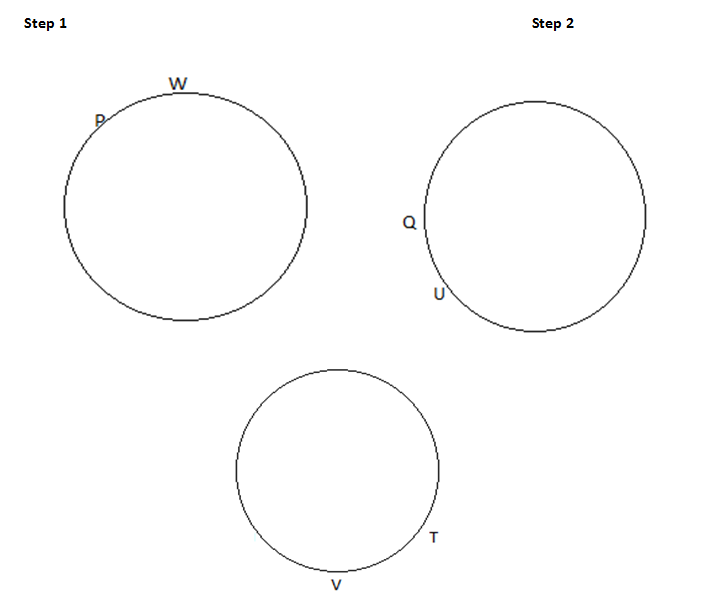Directions: P, Q, R, S, T, U, V & W are eight friends sitting in a circle facing towards the centre.
(i) W is to the immediate left of P but is not the neighbour of T and S.
(ii) U is to the immediate right of Q and V is a neighbour of T.
(iii) R is between T and U.

Question 1: What is the position of V?
(a) To the immediate right of W
(b) Between T and R
(c) Third to the right of U
(d) Second to the left of S
(e) None of these

Question 2: What is the position of S?
(a) Between Q and U
(b) To the immediate left of P
(c) Second to the right of U
(d) To the immediate left of Q
(e) None of these

Question 3: Which of the following statements is true?
(a) U is the neighbour of V
(b) V is between W and T
(c) W is between P and S
(d) T is between U and Q
(e) None of these

### Answers and Explanations: Click the down arrow to expand

From the given information, we can make the following diagram.

W is not a neighbour of T and SCommon Solution: From the above diagrams, we can make the following final diagram.Answer 1: (c) The position of V is third to the right of U. Correct option is (c).

Answer 2: (d) The correct position of S is to the immediate left of Q. Correct option is (d)

Answer 3: (b) From the given information, we can make the following diagram. Hence, V is between W and T.